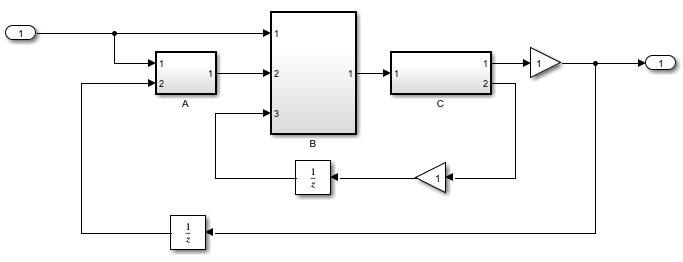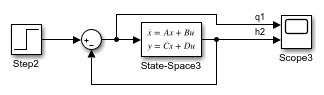## Remove Algebraic Loops

Use these techniques to remove algebraic loops in a model.

### Introduce a Delay to Remove Algebraic Loops

This example demonstrates how to remove algebraic loops in a model by introducing delays between blocks in a loop. This is one approach to remove algebraic loops in larger models where such loops can occur due to feedback between atomic subsystems.

Consider the model attached with this example. There are two algebraic loops caused by the atomic subsystems in the model

• `Blackbox A` -> `Blackbox B` -> `Blackbox C` -> `Blackbox A`

• `Blackbox B` -> `Blackbox C` -> `Blackbox B`When you update this model, Simulink® detects the loop `Blackbox A` -> `Blackbox B` -> `Blackbox C` -> `Blackbox A`

Since you do not know the contents of these subsystems, break the loops by adding a Unit Delay block outside the subsystems. There are three ways to use the Unit Delay block to break these loops:

• Add a Unit Delay between `Blackbox A` and `Blackbox C`.

• Add a Unit Delay between `Blackbox B` and `Blackbox C`.

• Add Unit Delay blocks to both algebraic loops.

Add a unit delay between `BlackBox A` and `BlackBox C`

If you add a unit delay on the feedback signal between the subsystems `Blackbox A` and `Blackbox C`, you introduce the minimum number of unit delays (1) to the system. By introducing the delay before `Blackbox A`, `Blackbox B` and `Blackbox C` use data from the current time step.Add a unit delay between `BlackBox B` and `BlackBox C`

If you add a unit delay between the subsystems `Blackbox B` and `Blackbox C`, you break the algebraic loop between `Blackbox B` and `Blackbox C`. In addition, you break the loop between `Blackbox A` and `Blackbox C`, because that signal completes the algebraic loop. By inserting the Unit Delay block before `Blackbox C`, `Blackbox C` now works with data from the previous time step only.Add unit delays to both algebraic loops

In the example here, you insert Unit Delay blocks to break both algebraic loops. In this model, BlackBox_A and BlackBox_B use data from the previous time step. BlackBox_C uses data from the current time step.### Solve Algebraic Loops Manually

If Simulink® cannot solve the algebraic loop, the software reports an error. Use one of these techniques to solve the loop manually:

• Restructure the underlying DAEs using techniques such as differentiation or change of coordinates. These techniques put the DAEs in a form that is easier for the algebraic loop solver to solve.

• Convert the DAEs to ODEs, which eliminates any algebraic loops.

#### Create Initial Guesses Using the IC and Algebraic Constraint Blocks

Your model might contain loops for which the loop solver cannot converge without a good, initial guess for the algebraic states. You can specify an initial guess for the algebraic state variables, but use this technique only when you think the loop is legitimate.

There are two ways to specify an initial guess:

• Place an IC block in the algebraic loop.

• Specify an initial guess for a signal in an algebraic loop using an Algebraic Constraint block.

### How Simulink Eliminates Artificial Algebraic Loops

When you enable Minimize algebraic loop occurrences, Simulink tries to eliminate artificial algebraic loops. In this example, the model contains an atomic subsystem that causes an artificial algebraic loop.The contents of the atomic subsystem are not direct feedthrough, but Simulink identifies the atomic subsystem as direct feedthrough.If the Algebraic loop diagnostic is set to `error`, simulating the model results in an error because the model contains an artificial algebraic loop involving its atomic subsystem.

To eliminate this algebraic loop,

1. Create the model from the preceding graphics, with the atomic subsystem that causes the artificial algebraic loop.

2. In the Diagnostics pane of Model Configuration Parameters, set the Algebraic loop parameter to `warning` or `none`.

3. To display the block execution order for this model and the atomic subsystem, in the Debug tab, select Information Overlays > Execution Order.

Reviewing the execution order can help you understand how to eliminate the artificial algebraic loop.

All the blocks in the subsystem execute at the same level: 1. (0 is the lowest level, indicating the first blocks to execute.)Note

4. In the top-level model’s Subsystem Parameters dialog box, select Minimize algebraic loop occurrences. This parameter directs the software to try to eliminate the algebraic loop that contains the atomic subsystem when you simulate the model.

5. Save the changes.

6. In the Modeling tab, click Update Model to recalculate the execution order.

Now there are two levels of execution order inside the subsystem: 1 and 2.To eliminate the artificial algebraic loop, the software tries to adjust the execution order so that the subsystem or model reference does not have direct feedthrough.

When you simulate a model, all blocks execute methods in this order:

1. `mdlOutputs`

2. `mdlDerivatives`

3. `mdlUpdate`

In the original version of this model, the execution of the `mdlOutputs` method starts with the Plant block because the Plant block does not have direct feedthrough. The execution finishes with the Controller block.If you enable the Minimize algebraic loop occurrences parameter for the atomic subsystem, the software divides the subsystem into two atomic units.These conditions are true:

• Atomic unit 2 does not have direct feedthrough.

• Atomic unit 1 has only a `mdlOutputs` method.

Only the `mdlDerivatives` or `mdlUpdate` methods of Atomic unit 2 need the output of Atomic unit 1. Simulink can execute what normally would have been executed during the `mdlOutput` method of Atomic unit 1 in the `mdlDerivatives` methods of Atomic unit 2.

The new execution order for the model is:

1. `mdlOutputs` method of model

1. `mdlOutputs` method of Atomic unit 2

2. `mdlOutputs` methods of other blocks

2. `mdlDerivatives` method of model

1. `mdlOutputs` method of Atomic unit 1

2. `mdlDerivatives` method of Atomic unit 2

3. `mdlDerivatives` method of other blocks

For the Minimize algebraic loop occurrences technique to be successful, the subsystem or referenced model must have a non-direct-feedthrough block connected directly to an Inport and must not contain any signals designated as test points. The software can then set the `DirectFeedthrough` property of the block Inport to `false` to indicate that the input port does not have direct feedthrough.

### Eliminate Artificial Algebraic Loops Caused by Atomic Subsystems

If an atomic subsystem causes an artificial algebraic loop, convert the atomic subsystem to a virtual subsystem. When the subsystem is atomic and you simulate the model, Simulink invokes the algebraic loop solver. The solver terminates after one iteration. The algebraic loop is automatically solved because there is no algebraic constant. After you make the subsystem virtual, Simulink does not invoke the algebraic loop solver during simulation.

To convert an atomic subsystem to a virtual subsystem:

1. Open the model that contains the atomic subsystem.

2. Right-click the atomic subsystem and select Subsystem Parameters.

3. Clear the Treat as atomic unit parameter.

4. Save the changes.

If you replace the atomic subsystem with a virtual subsystem and the simulation still fails with an algebraic loop error, examine the model for one of these:

• An algebraic constraint

• An artificial algebraic loop that was not caused by this atomic subsystem

### Bundled Signals That Create Artificial Algebraic Loops

Some models bundle signals together. This bundling can cause Simulink to detect an algebraic loop, even when an algebraic constraint does not exist. If you redirect one or more signals, you may be able to remove the artificial algebraic loop.

In this example, a linearized model simulates the dynamics of a two-tank system fed by a single pump. In this model:

• Output `q1` is the rate of the fluid flow into the tank from the pump.

• Output `h2` is the height of the fluid in the second tank.

• The State-Space block defines the dynamic response of the tank system to the pump operation:• The output from the State-Space block is a vector that contains `q1` and `h2`.If you simulate this model with the Algebraic loop parameter set to `warn` or `error`, Simulink identifies the algebraic loop.To eliminate this algebraic loop:

1. Change the `C` and `D` matrices as follows:2. Pass `q1` directly to the Scope instead of through the State-Space block.

Now, the input (`q1`) does not pass directly to the output (the `D` matrix is 0), so the State-Space block no longer has direct feedthrough. The feedback signal has only one element now, so the Selector block is no longer necessary, as you can see in the following model.### Model and Block Parameters to Diagnose and Eliminate Artificial Algebraic Loops

There are two parameters to consider when you think that your model has an artificial algebraic loop:

• Minimize algebraic loop occurrences parameter — Specify that Simulink try to eliminate any artificial algebraic loops for:

• Atomic subsystems — In the Subsystem Parameters dialog box, select Minimize algebraic loop occurrences.

• Model blocks — For the referenced model, in the Model Referencing pane of Configuration Parameters, select Minimize algebraic loop occurrences.

• Minimize algebraic loop parameter — Specifies what diagnostic action Simulink takes if the Minimize algebraic loop occurrences parameter has no effect.

The Minimize algebraic loop parameter is in the Diagnostics pane of Configuration Parameters. The diagnostic actions for this parameter are:

Setting Simulation Response
`none`

`warning`

Simulink displays a warning that the Minimize algebraic loop occurrences parameter has no effect.

`error`

Simulink terminates the simulation and displays an error that the Minimize algebraic loop occurrences parameter has no effect.

### Block Reduction and Artificial Algebraic Loops

When you enable the Block reduction optimization in Model Configuration Parameters, Simulink collapses certain groups of blocks into a single, more efficient block, or removes them entirely. Enabling block reduction results in faster execution during model simulation and in generating code.

Enabling block reduction can also help Simulink solve artificial algebraic loops.

Consider the following example model.Initially, block reduction is turned off. When you simulate this model, the Atomic Unit subsystem and Gain and Compare to Constant blocks are part of an algebraic loop that Simulink cannot solve.If you enable block reduction and execution order, and simulate the model again, Simulink does not display the execution order for blocks that have been reduced. You can now quickly see which blocks have been reduced.The Compare to Constant and Gain blocks have been eliminated from the model, so they no longer generate an algebraic loop error. The Atomic Unit subsystem generates a warning:

```Warning: If the inport 'ex_aloop_block_reduction_errwarn/ Atomic Unit/In1' of subsystem 'ex_aloop_block_reduction_errwarn/ Atomic Unit' involves direct feedback, then an algebraic loop exists, which Simulink cannot remove. Consider clearing the 'Minimize algebraic loop occurrences' parameter to avoid this warning.```

Tip

Use Bus Selector blocks to pass only the required signals into atomic subsystems.